## An alpha particle (Î±), which is the same as a helium-4 nucleus, is momentarily at rest in a region of space occupied by an electric field.

Question

An alpha particle (Î±), which is the same as a helium-4 nucleus, is momentarily at rest in a region of space occupied by an electric field. The particle then begins to move. Find the speed of the alpha particle after it has moved through a potential difference of â3.45Ã10^â3 V .The charge and the mass of an alpha particle are qÎ± = 3.20Ã10^â19 C and mÎ± = 6.68Ã10â27 kg , respectively.

Mechanical energy is conserved in the presence of which of the following types of forces?

a. electrostatic
b. frictional
c. magnetic
d. gravitational

in progress 0
5 months 2021-09-04T08:28:22+00:00 1 Answers 8 views 0

Speed = 575 m/s

Mechanical energy is conserved in electrostatic, magnetic and gravitational forces.

Explanation:

Given :

Potential difference, U =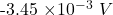Mass of the alpha particle,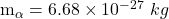Charge of the alpha particle is,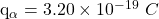So the potential difference for the alpha particle when it is accelerated through the potential difference is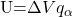And the kinetic energy gained by the alpha particle is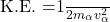From the law of conservation of energy, we get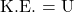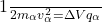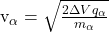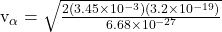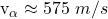The mechanical energy is conserved in the presence of the following conservative forces :

— electrostatic forces

— magnetic forces

— gravitational forces